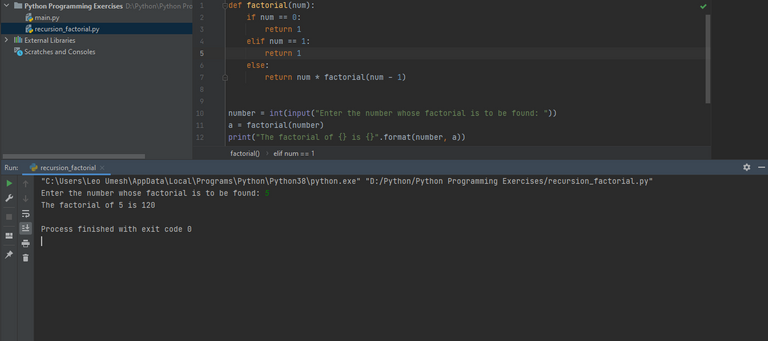# FINDING FACTORIAL OF A NUMBER USING RECURSION IN PYTHON

in OCD5 months ago

Recursion is a function that calls itself until a predefined condition is met. Recursion is an important concept in programming but should be used efficiently. Otherwise, setting improper conditions will make infinite recursion execute unless computer runs out of memory or procession power and the performance can be slow. In the below example, we will use a recursive function called factorial(num) that takes one parameter called num. Remember in order to find the factorial of 5 (i.e. 5! = 5*4!), we need to have factorial of 4 and in the same way, to find factorial of 4, we need to have factorial of 3 and so on. So, to find the factorial of a certain number, we need to apply factorial to its preceding numbers.

``````def factorial(num):
if num == 0:
return 1
elif num == 1:
return 1
else:
return num * factorial(num - 1)

number = int(input("Enter the number whose factorial is to be found: "))
a = factorial(number)
print("The factorial of {} is {}".format(number, a))
``````

Executing above code will give the following output.You can test this code online here.